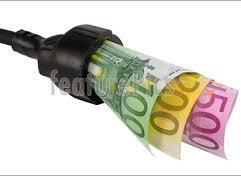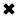# Electricity Cost Principles Explained#### Electricity Cost

Electricity Cost principles involve looking at how much electricity consumption and we have to understand how it's measured.

It is determined at any moment and is measured in watts consumed. For example: if you want to determine the bill energy or bill electricity rate:

• A 100-watt light bulb uses 100 watts.
• A typical desktop computer uses 65 watts.
• A central air conditioner uses about 3500 watts.

If you want to know how to rate electricity pricing, you want to know how much energy you're using. When you use 1000 watts for an hour, that's a kilowatt-hour. For example:

• Ten 100-watt light bulbs on for an hour, is 1 kWh
• Ten 100-watt light bulbs on for 1/2 an hour, is 0.5 kWh
• Ten 50-watt light bulbs on for an hour, is 0.5 kWh
• One 60-watt light bulb on for an hour, is 0.06 kWh (60/1000)
• Running a 3500-watt air conditioner for an hour is 3.5 kWh.

The average U.S. household used 10,654 kWh a year in 2001, or 888 kWh/mo. (Dept. of Energy) The U.S. as a whole used 3,883 billion kWh in 2003, or 13,868 kwH per person based on a population of 300 million. (Dept. of Energy)

Watt-hours

For smaller items we use the term watt-hours instead of kilowatt-hours. For example, we say a 60-watt light bulb uses 60 watt-hours of electricity billed, not 0.060 kWh.

Note that the "-hours" part is important. Without it we'd have no idea what period of time we were talking about.

If you ever see a reference without the amount of time specified, it's almost certainly per hour.

If your device lists amps instead of watts, then just multiply the amps times the voltage to get the watts. For example:

2.5 amps x 120 volts = 300 watts

Trivia: On a peak day in 2009, California used 50,743 megawatt-hours of electricity, or 50,743,000,000 watt-hours.

How much does electricity cost?

Electricity Cost

It depends on where you live (like Ontario), how much you use, and possibly when you use it. There are also fixed charges that you pay every month no matter how much electricity you use. For example, I pay \$6/mo. for the privilege of being a customer of the electric company, no matter how much energy I use.

Check your utility bill for the rates in your area. If it's not on your bill then look it up on the utility's website.

The electric company measures how much electricity you use in kilowatt-hours. The abbreviation for killowatt-hour is kWh. Note that on your bill there can be multiple charges per kWh (e.g., one for the "base rate", another for "fuel") and you have to add them all up to get the total cost per kWh.

Most utility companies charge a higher rate when you use more than a certain amount of energy, and they also charge more during summer months when electric use is higher. As an example, here are the residential rates prices electricity for Austin, Texas (as of 11-03):

First 500 kilowatts5.8¢ per kilowatt hour (kWh)

Additional kilowatts (May-Oct.)10¢ per kilowatt hour

Additonal kilowatts (Nov.-Apr.)8.3¢ per kilowatt hour

These figures include a fuel charge of 2.265¢ per kWh.

The average cost of residential electricity was 9.86¢/kWh in the U.S. in March 2006. The average household used 888 kWh/mo. in 2001 and would pay \$87.56 for it based on the March 2006 average rate. (Dept. of Energy)

The cost of electricity varies by region. In 2003 the price ranged from 5.81¢ in Tennessee to 12¢ in California, 14.314¢ in New York, and 16.734¢ in Hawaii. In Summer 2001, electricity was a whopping 20¢/kWh in parts of California.

##### Basic Electricity Handbook, Vol. 1• FULLY ILLUSTRATED
• GREAT FOR SCHOOL PROJECTS
• GREAT PRICE: \$5.99
• ...

This 100+ page e-book is a great guide for those who have a basic interest in the field of electricity. This well-illustrated e-book, coupled with some basic knowledge of electricity, will give you a broad theoretical background in this fundamental subject.

CONTENTS
• FUNDAMENTALS OF ELECTRICITY
• HOW DOES ELECTRICITY WORK?
• HOW IS ELECTRICITY GENERATED?
• WHERE DOES ELECTRICITY COME FROM?
• BASIC HOME WIRING
• ELECTRICITY FOR STUDENTS
• ENERGY SAVING TIPS

##### TRAINING EF COURSES

Content Community Connection
Top